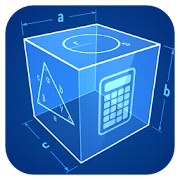# Geometry CalculatorEveryone
224
Geometric Calculator

Calculates plane and solid figures:
Triangle, square, rectangle, parallelogram, rhombus, trapezoid, rectangle, polygon, circle, circle, ellipse.
Sphere, cube, box, cylinder, cone, truncated cone, prism, pyramid, truncated pyramid, the octahedron.

For every geometry shape there is step by step math solve solution provided! If you either a schoolkid or an undergraduate this math solver toolkit will be helpful for you!

Planimetrics:
~~~~~~~~~~
Triangle
- Parties
- angles
- Area
- Perimeter

square
- Parties
- diagonal
- Area
- Perimeter

rectangle
- diagonal
- Area
- Perimeter

parallelogram
- Diagonals
- Area
- Perimeter

rhombus
- Diagonals
- Area
- Perimeter

trapeze
- Area
- Perimeter

- Area
- Perimeter

polygon
- angles
- Area

circle
- Area
- The length of the arc
- Size of the sector
- The length of the arc segment
- The area of the segment

ring
- Area
- Size of the sector

ellipse
- Area
- Perimeter

Solid geometry:
~~~~~~~~~~~~

ball
- Area
- Volume

cube
- Area
- Volume

parallelepiped
- Area
- Volume

cylinder
- Area
- Volume
- The area of the lateral surface

cone
- Area
- Volume
- The area of the lateral surface

frustum
- Area
- Volume
- The area of the lateral surface

prism
- Area
- Volume

pyramid
- Area
- Volume
- The area of the lateral surface

truncated pyramid
- Volume

octahedron
- Area
- Volume
Collapse

Review Policy
4.3
224 total
5
4
3
2
1

## What's New

- Bug with the imposition of figures is fixed
- The minimum size of fonts is increased
Collapse

Eligible for Family LibraryEligible if bought after 7/2/2016. Learn More
Updated
July 25, 2019
Size
3.8M
Installs
1,000+
Current Version
2.8
Requires Android
4.1 and up
Content Rating
Everyone
Permissions
Offered By
ARPAPLUS
Developer